# Verilog Code for 16-bit RISC Processor

## In this Verilog project, Verilog code for a 16-bit RISC processor is presented.

### The RISC processor is designed based on its instruction set and Harvard-type data path structure. Then, the RISC processor is implemented in Verilog and verified using Xilinx ISIM.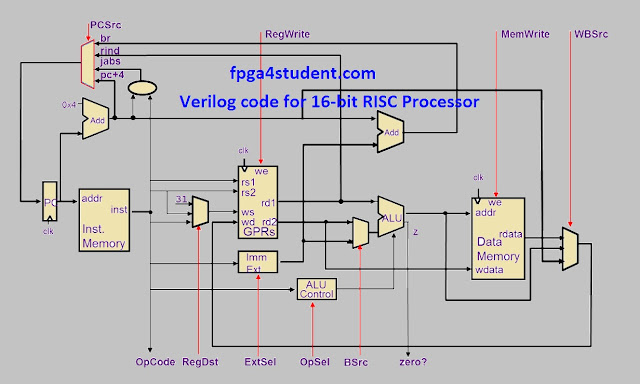#### The instruction set of the RISC processor:

A. Memory Access Instructions
LD ws, offset(rs1) ws:=Mem16[rs1 + offset]
2. Store Word:
ST rs2, offset(rs1) Mem16[rs1 + offset]=rs2

B. Data Processing Instructions
ADD ws, rs1, rs2 ws:=rs1 + rs2
2. Subtract:
SUB ws, rs1, rs2 ws:=rs1 – rs2
3. Invert (1‘s complement):
INV ws, rs1 ws:=!rs1
4. Logical Shift Left:
LSL ws, rs1, rs2 ws:=rs1 << rs2
5. Logical Shift Right:
LSR ws, rs1, rs2 ws:=rs1 >> rs2
6. Bitwise AND:
AND ws, rs1, rs2 ws:=rs1 • rs2
7. Bitwise OR:
OR ws, rs1, rs2 ws:=rs1 | rs2
8. Set on Less Than:
SLT ws, rs1, rs2 ws:=1 if rs1 < rs2; ws:=0 if rs1 ≥ rs2

C. Control Flow Instructions
1. Branch on Equal:
BEQ rs1, rs2, offset
Branch to (PC + 2 + (offset << 1)) when rs1 = rs2

2. Branch on Not Equal:
BNE rs1, rs2, offset
Branch to (PC + 2 + (offset << 1)) when rs1 != rs2

#### Instruction Format of the RISC processor :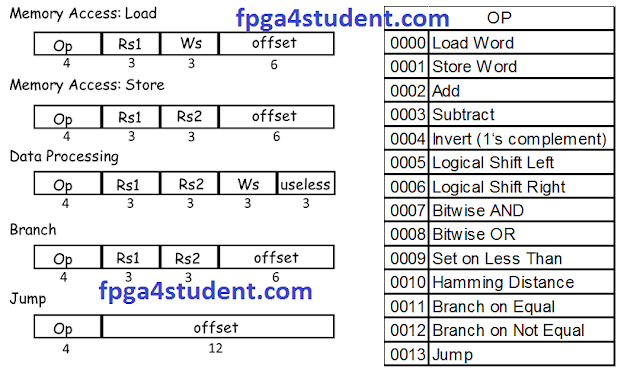#### Processor Control Unit Design:

 Control signals Instruction Reg Dst ALUSrc Memto Reg Reg Write MemRead Mem Write Branch ALUOp Jump Data-processing 1 0 0 1 0 0 0 00 0 LW 0 1 1 1 1 0 0 10 0 SW 0 1 0 0 0 1 0 10 0 BEQ,BNE 0 0 0 0 0 0 1 01 0 J 0 0 0 0 0 0 0 00 1

#### ALU Control Unit Design:

 ALU Control ALUOp Opcode(hex) ALUcnt ALU Operation Instruction 10 xxxx 000 ADD LW,SW 01 xxxx 001 SUB BEQ,BNE 00 0002 000 ADD D-type: ADD 00 0003 001 SUB D-type: SUB 00 0004 010 INVERT D-type: INVERT 00 0005 011 LSL D-type: LSL 00 0006 100 LSR D-type: LSR 00 0007 101 AND D-type: AND 00 0008 110 OR D-type: OR 00 0009 111 SLT D-type: SLT

### Verilog code for the RISC processor:

#### 1. Verilog code for Instruction Memory :

````include "Parameter.v"
// fpga4student.com
// FPGA projects, VHDL projects, Verilog projects
// Verilog code for RISC Processor
// Verilog code for Instruction Memory
module Instruction_Memory(
input[15:0] pc,
output[15:0] instruction
);

reg [`col - 1:0] memory [`row_i - 1:0];
wire [3 : 0] rom_addr = pc[4 : 1];
initial
begin
end

endmodule
```

#### 2. Verilog code for register file:

````timescale 1ns / 1ps
// fpga4student.com
// FPGA projects, VHDL projects, Verilog projects
// Verilog code for RISC Processor
// Verilog code for register file
module GPRs(
input    clk,
// write port
input    reg_write_en,
input  [2:0] reg_write_dest,
input  [15:0] reg_write_data,
);
reg [15:0] reg_array [7:0];
integer i;
// write port
//reg [2:0] i;
initial begin
for(i=0;i<8;i=i+1)
reg_array[i] <= 16'd0;
end
always @ (posedge clk ) begin
if(reg_write_en) begin
reg_array[reg_write_dest] <= reg_write_data;
end
end

endmodule
```

#### 3. Verilog code for Data Memory:

````include "Parameter.v"
// fpga4student.com
// FPGA projects, VHDL projects, Verilog projects
// Verilog code for RISC Processor
// Verilog code for data Memory
module Data_Memory(
input clk,

// write port
input [15:0]   mem_write_data,
input     mem_write_en,
);

reg [`col - 1:0] memory [`row_d - 1:0];
integer f;
initial
begin

f = \$fopen(`filename);
\$fmonitor(f, "time = %d\n", \$time,
"\tmemory = %b\n", memory,
"\tmemory = %b\n", memory,
"\tmemory = %b\n", memory,
"\tmemory = %b\n", memory,
"\tmemory = %b\n", memory,
"\tmemory = %b\n", memory,
"\tmemory = %b\n", memory,
"\tmemory = %b\n", memory);
`simulation_time;
\$fclose(f);
end

always @(posedge clk) begin
if (mem_write_en)
end

endmodule
```

#### 4. Verilog code for ALU unit:

```// fpga4student.com
// FPGA projects, VHDL projects, Verilog projects
// Verilog code for RISC Processor
// Verilog code for ALU
module ALU(
input  [15:0] a,  //src1
input  [15:0] b,  //src2
input  [2:0] alu_control, //function sel

output reg [15:0] result,  //result
output zero
);

always @(*)
begin
case(alu_control)
3'b000: result = a + b; // add
3'b001: result = a - b; // sub
3'b010: result = ~a;
3'b011: result = a<<b;
3'b100: result = a>>b;
3'b101: result = a & b; // and
3'b110: result = a | b; // or
3'b111: begin if (a<b) result = 16'd1;
else result = 16'd0;
end
default:result = a + b; // add
endcase
end
assign zero = (result==16'd0) ? 1'b1: 1'b0;

endmodule
```

#### 5. Verilog code for ALU Control Unit of the RISC processor:

````timescale 1ns / 1ps
//fpga4student.com: FPGA projects, Verilog projects, VHDL projects
// Verilog code for 16-bit RISC processor
// ALU_Control Verilog code
module alu_control( ALU_Cnt, ALUOp, Opcode);
output reg[2:0] ALU_Cnt;
input [1:0] ALUOp;
input [3:0] Opcode;
wire [5:0] ALUControlIn;
assign ALUControlIn = {ALUOp,Opcode};
always @(ALUControlIn)
casex (ALUControlIn)
6'b10xxxx: ALU_Cnt=3'b000;
6'b01xxxx: ALU_Cnt=3'b001;
6'b000010: ALU_Cnt=3'b000;
6'b000011: ALU_Cnt=3'b001;
6'b000100: ALU_Cnt=3'b010;
6'b000101: ALU_Cnt=3'b011;
6'b000110: ALU_Cnt=3'b100;
6'b000111: ALU_Cnt=3'b101;
6'b001000: ALU_Cnt=3'b110;
6'b001001: ALU_Cnt=3'b111;
default: ALU_Cnt=3'b000;
endcase
endmodule
```

### 6. Verilog code for Datapath of the RISC processor:

````timescale 1ns / 1ps
// fpga4student.com
// FPGA projects, VHDL projects, Verilog projects
// Verilog code for RISC Processor
// Verilog code for Data Path of the processor
module Datapath_Unit(
input clk,
input[1:0] alu_op,
output[3:0] opcode
);
reg  [15:0] pc_current;
wire [15:0] pc_next,pc2;
wire [15:0] instr;
wire [2:0] reg_write_dest;
wire [15:0] reg_write_data;
wire [2:0] ALU_Control;
wire [15:0] ALU_out;
wire zero_flag;
wire [15:0] PC_j, PC_beq, PC_2beq,PC_2bne,PC_bne;
wire beq_control;
wire [12:0] jump_shift;
// PC
initial begin
pc_current <= 16'd0;
end
always @(posedge clk)
begin
pc_current <= pc_next;
end
assign pc2 = pc_current + 16'd2;
// instruction memory
Instruction_Memory im(.pc(pc_current),.instruction(instr));
// jump shift left 2
assign jump_shift = {instr[11:0],1'b0};
// multiplexer regdest
assign reg_write_dest = (reg_dst==1'b1) ? instr[5:3] :instr[8:6];
// register file

// GENERAL PURPOSE REGISTERs
GPRs reg_file
(
.clk(clk),
.reg_write_en(reg_write),
.reg_write_dest(reg_write_dest),
.reg_write_data(reg_write_data),
);
// immediate extend
assign ext_im = {{10{instr}},instr[5:0]};
// ALU control unit
alu_control ALU_Control_unit(.ALUOp(alu_op),.Opcode(instr[15:12]),.ALU_Cnt(ALU_Control));
// multiplexer alu_src
// ALU
assign PC_beq = pc2 + {ext_im[14:0],1'b0};
assign PC_bne = pc2 + {ext_im[14:0],1'b0};
// beq control
assign beq_control = beq & zero_flag;
assign bne_control = bne & (~zero_flag);
// PC_beq
assign PC_2beq = (beq_control==1'b1) ? PC_beq : pc2;
// PC_bne
assign PC_2bne = (bne_control==1'b1) ? PC_bne : PC_2beq;
// PC_j
assign PC_j = {pc2[15:13],jump_shift};
// PC_next
assign pc_next = (jump == 1'b1) ? PC_j :  PC_2bne;

/// Data memory
Data_Memory dm
(
.clk(clk),
.mem_write_en(mem_write),
);

// write back
assign reg_write_data = (mem_to_reg == 1'b1)?  mem_read_data: ALU_out;
// output to control unit
assign opcode = instr[15:12];
endmodule
```

### 7. Verilog code for the Control Unit of the RISC processor:

````timescale 1ns / 1ps
// fpga4student.com
// FPGA projects, VHDL projects, Verilog projects
// Verilog code for RISC Processor
// Verilog code for Control Unit
module Control_Unit(
input[3:0] opcode,
output reg[1:0] alu_op,
);

always @(*)
begin
case(opcode)
4'b0000:  // LW
begin
reg_dst = 1'b0;
alu_src = 1'b1;
mem_to_reg = 1'b1;
reg_write = 1'b1;
mem_write = 1'b0;
beq = 1'b0;
bne = 1'b0;
alu_op = 2'b10;
jump = 1'b0;
end
4'b0001:  // SW
begin
reg_dst = 1'b0;
alu_src = 1'b1;
mem_to_reg = 1'b0;
reg_write = 1'b0;
mem_write = 1'b1;
beq = 1'b0;
bne = 1'b0;
alu_op = 2'b10;
jump = 1'b0;
end
4'b0010:  // data_processing
begin
reg_dst = 1'b1;
alu_src = 1'b0;
mem_to_reg = 1'b0;
reg_write = 1'b1;
mem_write = 1'b0;
beq = 1'b0;
bne = 1'b0;
alu_op = 2'b00;
jump = 1'b0;
end
4'b0011:  // data_processing
begin
reg_dst = 1'b1;
alu_src = 1'b0;
mem_to_reg = 1'b0;
reg_write = 1'b1;
mem_write = 1'b0;
beq = 1'b0;
bne = 1'b0;
alu_op = 2'b00;
jump = 1'b0;
end
4'b0100:  // data_processing
begin
reg_dst = 1'b1;
alu_src = 1'b0;
mem_to_reg = 1'b0;
reg_write = 1'b1;
mem_write = 1'b0;
beq = 1'b0;
bne = 1'b0;
alu_op = 2'b00;
jump = 1'b0;
end
4'b0101:  // data_processing
begin
reg_dst = 1'b1;
alu_src = 1'b0;
mem_to_reg = 1'b0;
reg_write = 1'b1;
mem_write = 1'b0;
beq = 1'b0;
bne = 1'b0;
alu_op = 2'b00;
jump = 1'b0;
end
4'b0110:  // data_processing
begin
reg_dst = 1'b1;
alu_src = 1'b0;
mem_to_reg = 1'b0;
reg_write = 1'b1;
mem_write = 1'b0;
beq = 1'b0;
bne = 1'b0;
alu_op = 2'b00;
jump = 1'b0;
end
4'b0111:  // data_processing
begin
reg_dst = 1'b1;
alu_src = 1'b0;
mem_to_reg = 1'b0;
reg_write = 1'b1;
mem_write = 1'b0;
beq = 1'b0;
bne = 1'b0;
alu_op = 2'b00;
jump = 1'b0;
end
4'b1000:  // data_processing
begin
reg_dst = 1'b1;
alu_src = 1'b0;
mem_to_reg = 1'b0;
reg_write = 1'b1;
mem_write = 1'b0;
beq = 1'b0;
bne = 1'b0;
alu_op = 2'b00;
jump = 1'b0;
end
4'b1001:  // data_processing
begin
reg_dst = 1'b1;
alu_src = 1'b0;
mem_to_reg = 1'b0;
reg_write = 1'b1;
mem_write = 1'b0;
beq = 1'b0;
bne = 1'b0;
alu_op = 2'b00;
jump = 1'b0;
end
4'b1011:  // BEQ
begin
reg_dst = 1'b0;
alu_src = 1'b0;
mem_to_reg = 1'b0;
reg_write = 1'b0;
mem_write = 1'b0;
beq = 1'b1;
bne = 1'b0;
alu_op = 2'b01;
jump = 1'b0;
end
4'b1100:  // BNE
begin
reg_dst = 1'b0;
alu_src = 1'b0;
mem_to_reg = 1'b0;
reg_write = 1'b0;
mem_write = 1'b0;
beq = 1'b0;
bne = 1'b1;
alu_op = 2'b01;
jump = 1'b0;
end
4'b1101:  // J
begin
reg_dst = 1'b0;
alu_src = 1'b0;
mem_to_reg = 1'b0;
reg_write = 1'b0;
mem_write = 1'b0;
beq = 1'b0;
bne = 1'b0;
alu_op = 2'b00;
jump = 1'b1;
end
default: begin
reg_dst = 1'b1;
alu_src = 1'b0;
mem_to_reg = 1'b0;
reg_write = 1'b1;
mem_write = 1'b0;
beq = 1'b0;
bne = 1'b0;
alu_op = 2'b00;
jump = 1'b0;
end
endcase
end

endmodule
```

### 8. Verilog code for the 16-bit RISC processor:

````timescale 1ns / 1ps
// fpga4student.com
// FPGA projects, VHDL projects, Verilog projects
// Verilog code for RISC Processor

module Risc_16_bit(
input clk
);
wire[1:0] alu_op;
wire [3:0] opcode;
// Datapath
Datapath_Unit DU
(
.clk(clk),
.jump(jump),
.beq(beq),
.mem_write(mem_write),
.alu_src(alu_src),
.reg_dst(reg_dst),
.mem_to_reg(mem_to_reg),
.reg_write(reg_write),
.bne(bne),
.alu_op(alu_op),
.opcode(opcode)
);
// control unit
Control_Unit control
(
.opcode(opcode),
.reg_dst(reg_dst),
.mem_to_reg(mem_to_reg),
.alu_op(alu_op),
.jump(jump),
.bne(bne),
.beq(beq),
.mem_write(mem_write),
.alu_src(alu_src),
.reg_write(reg_write)
);

endmodule
```

### 9. Verilog Testbench code for the 16-bit RISC Processor:

````timescale 1ns / 1ps
`include "Parameter.v"
// fpga4student.com
// FPGA projects, VHDL projects, Verilog projects
// Verilog code for RISC Processor
// Verilog testbench code to test the processor
module test_Risc_16_bit;

// Inputs
reg clk;

// Instantiate the Unit Under Test (UUT)
Risc_16_bit uut (
.clk(clk)
);

initial
begin
clk <=0;
`simulation_time;
\$finish;
end

always
begin
#5 clk = ~clk;
end

endmodule
```

#### Parameter file:

````ifndef PARAMETER_H_
`define PARAMETER_H_
// fpga4student.com
// FPGA projects, VHDL projects, Verilog projects
// Verilog code for RISC Processor
// Parameter file
`define col 16 // 16 bits instruction memory, data memory
`define row_i 15 // instruction memory, instructions number, this number can be changed. Adding more instructions to verify your design is a good idea.
`define row_d 8 // The number of data in data memory. We only use 8 data. Do not change this number. You can change the value of each data inside test.data file. Total number is fixed at 8.
`define filename "./test/50001111_50001212.o"
`define simulation_time #160

`endif
```

All Verilog code needed for the 16-bit RISC processor are provided. Now, you just need to create a test.data (Initial content of data memory) and test.prog (Intruction memory). Then, run simulation to see how the process works on simulation waveform and memory files.

#### Example instruction memory file:

```0000_0100_0000_0000 // load R0 <- Mem(R2 + 0)
0000_0100_0100_0001 // load R1 <- Mem(R2 + 1)
0010_0000_0101_0000 // Add R2 <- R0 + R1
0001_0010_1000_0000 // Store Mem(R1 + 0) <- R2
0011_0000_0101_0000 // sub R2 <- R0 - R1
0100_0000_0101_0000 // invert R2 <- !R0
0101_0000_0101_0000 // logical shift left R2 <- R0<<R1
0110_0000_0101_0000 // logical shift right R2 <- R0>>R1
0111_0000_0101_0000 // AND R2<- R0 AND R1
1000_0000_0101_0000 // OR R2<- R0 OR R1
1001_0000_0101_0000 // SLT R2 <- 1 if R0 < R1
0010_0000_0000_0000 // Add R0 <- R0 + R0
1011_0000_0100_0001 // BEQ branch to jump if R0=R1, PCnew= PC+2+offset<<1 = 28 => offset = 1
1100_0000_0100_0000 // BNE branch to jump if R0!=R1, PCnew= PC+2+offset<<1 = 28 => offset = 0

#### Example data memory file:

```0000_0000_0000_0001
0000_0000_0000_0010
0000_0000_0000_0001
0000_0000_0000_0010
0000_0000_0000_0001
0000_0000_0000_0010
0000_0000_0000_0001
0000_0000_0000_0010
```

1.ALU_Control_unit module is missing !

1.Updated. Thanks.

2.Description of ALU and ALU Control is still missing in the Datapath.

3.Check the given table to understand the functionality of ALU and ALU control unit.

4.to implent it on fpga module like spartan,can you please give a heads up

5.can yo just explain about the test.prog and test.data ,where should we create it and also about the .o file.

1.These files were given above. Just create the file with the same names and copy the contents into these files.

6.can u pls send me this code
my mail is

7.Can u send 8bit RISC processor code??

8.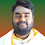Thank you for the above code. I tested it , works perfectly. I got an overview now. Thanks again.

1.Glad to hear that. You're welcome.

2.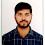Can you please send the codes beacuse i have tested and it was showing some errors the tool i have used is xilinx 14.3 version and still not showing the output

9.how to create a test.data (Initial content of data memory) and test.prog (Intruction memory).

1.For the instruction memory, you need to convert instructions to machine code. Then, put the data into instruction memory. There are 2 given example files for data and instruction memory. You can refer to it.

10.i did not get the output could you please tell me how to exactly execute it

1.Check register and memory content for verification or you take some of them as outputs to see on the simulation waveform

11.Can you please provide FSM for each blocks.

12.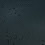Thanks for the post

13.Can u send me the code for 5stage pipeline 64 bit Risc processor with 32 instructions

14.How to create test.data and test.prog files and where should we create it?
Should they be a text file?

15.I am new in verilog, using Quartus II for simulation. Facing syntax error in test.prog but i don't get it where is the error. I have copied your given example as it is and saved it naming test.prog

16.Implementation in vivado says the design is empty. How to solve it ?

17.Could you explain the abbreviation of rs1,rs2 and ws

18.Sir, Can you tell me which simulator you used? I tried xilinx vivado... but I didnt understand where to check the output for that .data and .prog files.

1.it can be used for any Verilog simulator. You need to create the .data + .prog files in 'test' folder inside the project folder.

19.Can you please explain why in Instruction memory part in line
wire [3 : 0] rom_addr = pc[4 : 1];
you have not assigned pc[3:0]? why u hv left out 0th bit pc while assigning?

1.Each instruction/memory data width has 16 bits or 2 bytes. PC counted by 2. That's why only PC[4:1] is used to fetch instruction from ROM.